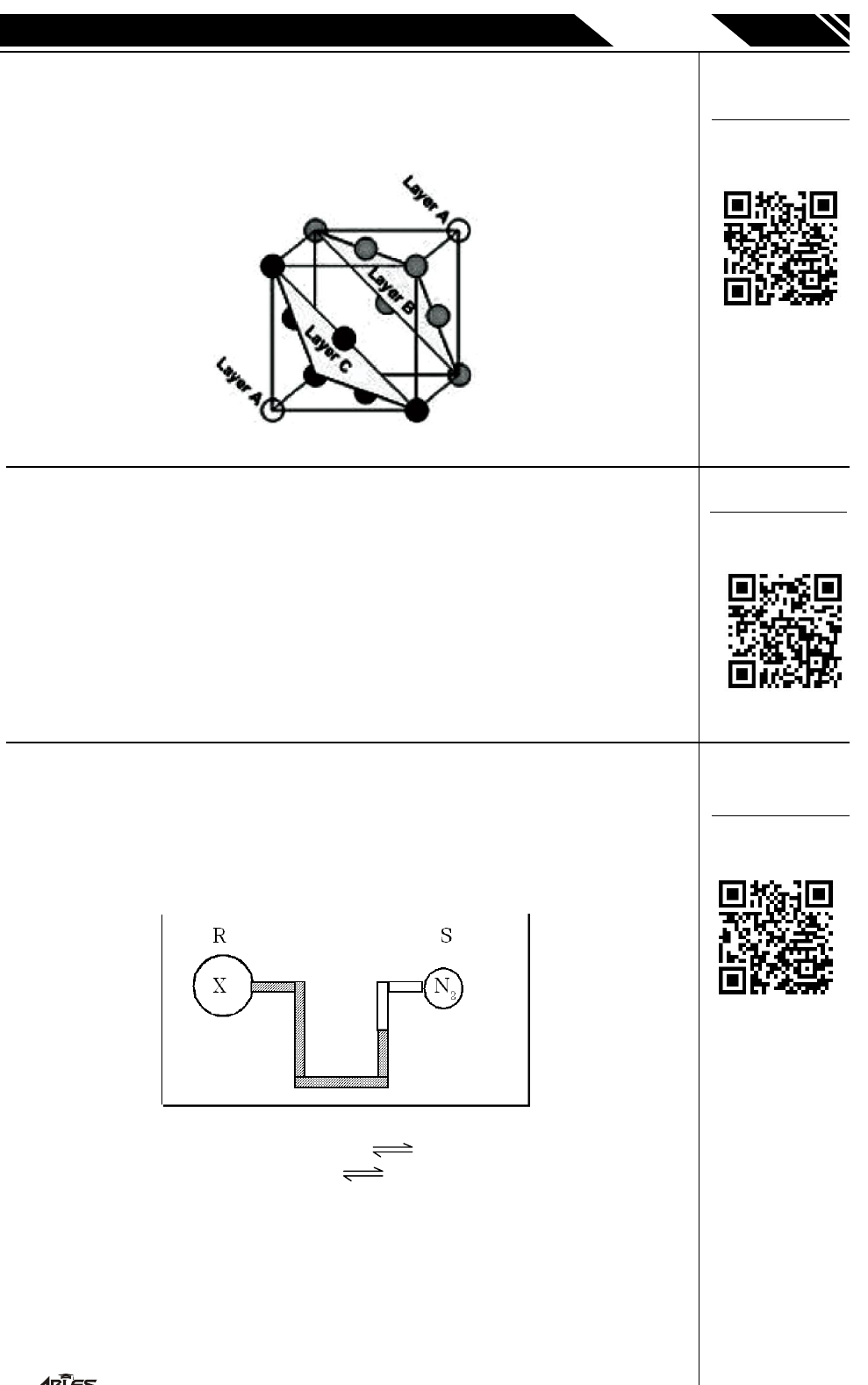IIT-JEE
CHEMISTRY
P. JOY
PROMOTIONAL MATTER
1
,
588-A, TAL
W
ANDI, KOT
A
(
Q.1 A metal crystallises into a lattice containing a sequence of
layers of ABC ABC ........... . If the radius of metal atoms
is 174 pm, then the distance between the two successive
layers (i.e., A and B) is-
(A) 348 pm (B) 174 pm (C) 284.2 pm (D) 492.2 pm
Q.2 Barium titanate has the perovskit structure which is a cubic
lattice, with barium ions occupying the corners of the unit
cell, oxide ions the face centers and titanium ions the
centres. Assuming that Ti
4+
ions are occupying the holes
of the BaO lattice, what type of holes and what fraction of
such holes does the ion occupy ?
(A) 50% Tetrahedral holes
(B) 25% Octahedral holes
(C) 100% Octahedral holes
(D) 25% Tetrahedral holes
Q.3 The two bulbs R and S connected by a mercury manometer
are held in a thermostat (to maintain constant temp.) Volume
of R is twice the volume of S. R contains a gas X at the same
pressure of nitrogen as in S. The mercury level in the right
ha nd limbrises whe n temperature is increased if th e gas X is
(A) Nitrogen
(B) An equilibrium mixture CH
3
NC(g)
CH
3
NC(g)
DH is negative.
(C) An equilibrium mixture N
2
F(g)
2NF(g)
DH is positive.
(D) Hydrogen.
To open video solution go to
https://ables.online/20video
or
Scan following QR Code
To open video solution go to
https://ables.online/20video
or
Scan following QR Code
To open video solution go to
https://ables.online/20video
or
Scan following QR Code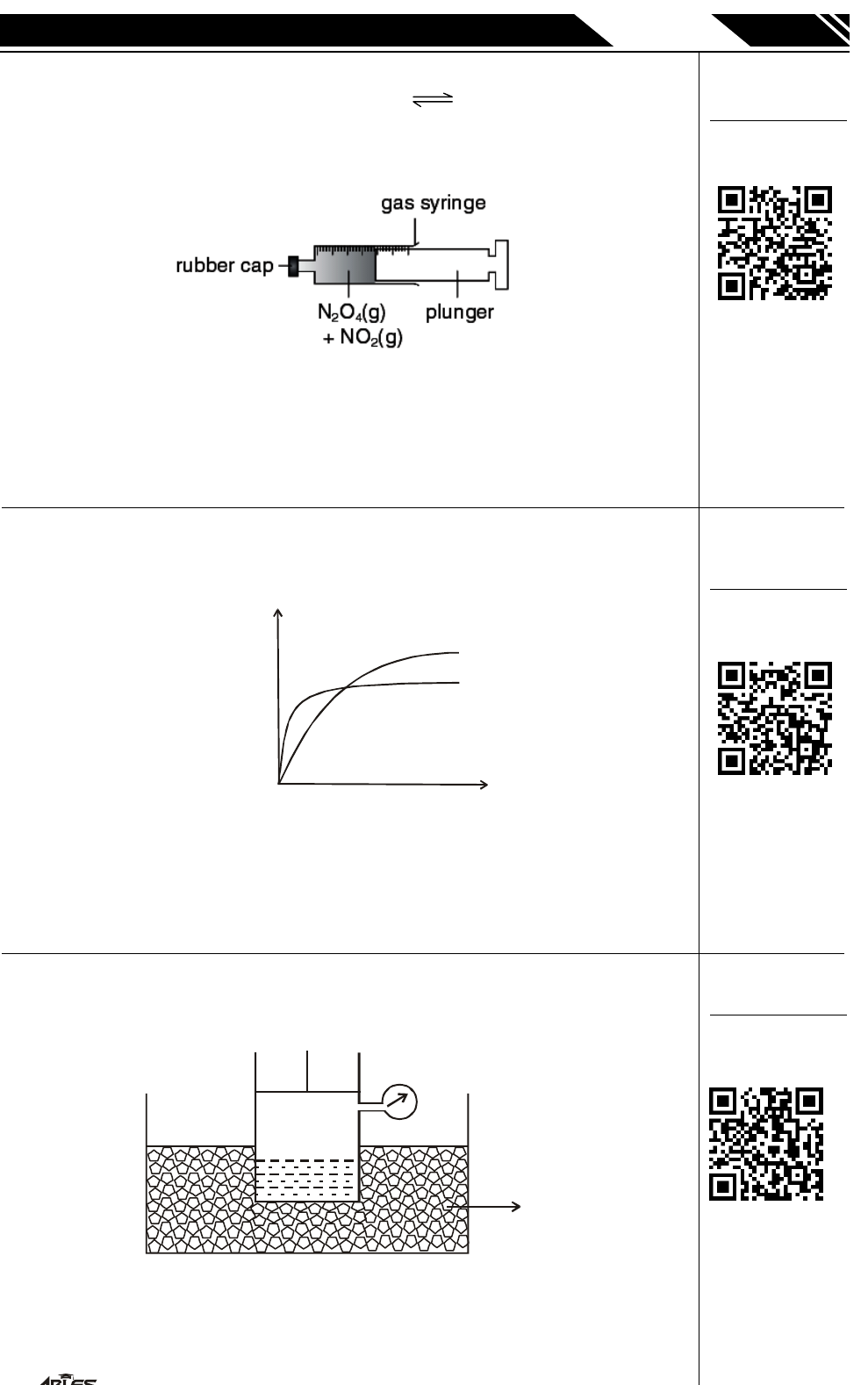IIT-JEE
CHEMISTRY
P. JOY
PROMOTIONAL MATTER
2
,
588-A, TAL
W
ANDI, KOT
A
(
Q.4 At room temperature, N
2
O
4
(g) and NO
2
(g) exist in
equilibrium as follows : N
2
O
4
(g)
2NO
2
(g)
pale yellow dark brown
At gas syringe is filled with a pale brown mixture of N
2
O
4
(g)
and NO
2
(g) at equilibrium.
What would be observed when the plunger is quickly pushed
in at time t ?
(A) The mixture first lightens, and then becomes colourless.
(B) The mixture first darkens, and then becomes colourless.
(C) The mixture first darkens, and then lightens.
(D) The mixture first lightens, and then darkens.
Q.5 In the diagram, curve X was obtained by observing the
decomposition of 100 cm
3
of 1.0 mol dm
–3
hydrogen
perioxide, catalysed by manganese (IV) oxide
(X)
(Y)
Time
Volume of
oxygen
formed
Which alteration to the original experimental conditions
would produce curve Y ?
(B) Adding some 0.1 mol dm
–3
hydrogen perioxide
(C) Using less manganese (IV) hydrogen peroxide
(D) Lowering the temperature
Q.6 Consider the given system. Vessel contains 50 gm water at
273 K. It contains air saturated with water vapour at 1200
mm Hg.
1200 mm Hg
Ice bath
What will be the final pressure in the vessel after the
volume of vessel has been changed to one third (vapour
pressure of water at this temperature 400 torr)
(A) 3600 mm (B) 2800 mm (C) 40 mm (D) 1200 mm
To open video solution go to
https://ables.online/20video
or
Scan following QR Code
To open video solution go to
https://ables.online/20video
or
Scan following QR Code
To open video solution go to
https://ables.online/20video
or
Scan following QR Code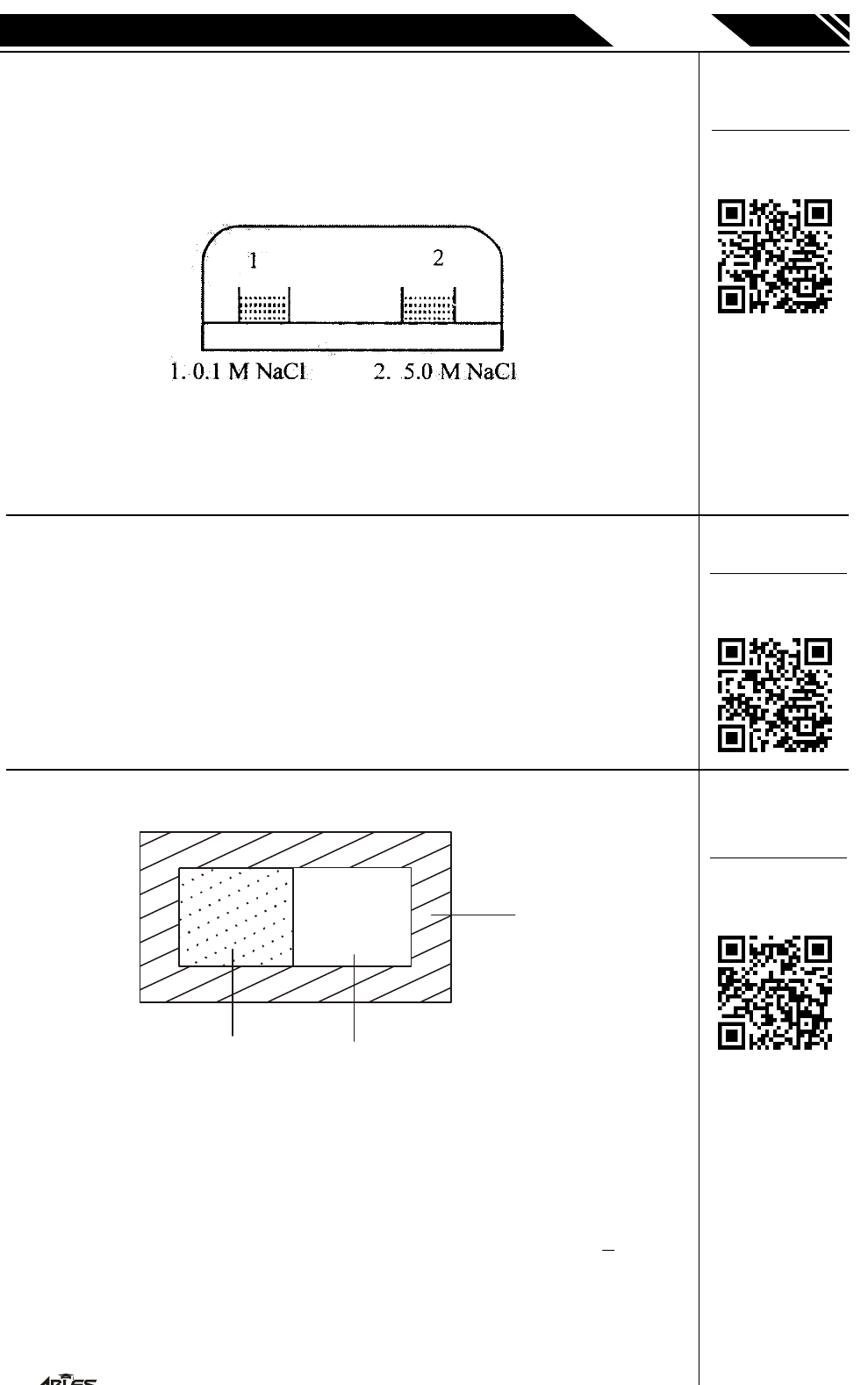IIT-JEE
CHEMISTRY
P. JOY
PROMOTIONAL MATTER
3
,
588-A, TAL
W
ANDI, KOT
A
(
Q.7 Equal volumes of 0.1M solution of NaCl and 5M solution of
NaCl are kept in a closed vessel (see figure) and
temperature is maintained constant. After equilibrium has
been established, assuming that volumes are additive, which
of the following is (are) true ?
(A) Volume of 0.1 M solution is lesser than its original volume
(B) Volume of 0.1M solution is greater than its original volume
(C) Volume of 5M solution is lesser than its original volume
(D) Volume of 5M solution is greater than its original volume
(E) No change occurs at all
Q.8 Insulin is dissolved in a suitable solvent and the osmotic
pressure (p) of solutions of various concentrations (g/cm
3
)
C is measured at 20ºC. The slope of a plot of p against C is
found to be 4.65 × 10
–3
. The molecular weight of the insulin
is :
(A) 3 × 10
5
(B) 9 × 10
5
(C) 4.5 × 10
5
(D) 5.16 × 10
6
Q.9
V Lit.
v Lit.
Thermal
insulator
'n' mole of an ideal gas is confined in V lit. volume, by
removing the partition gas is allowed to occupy V lit. of
vacuum. The temp. of gas is T and work done in pulling the
partition is 'O'. The change in entropy DS will be-
(A) 0 (B) nR ln 2
(C) nR ln 2 (D) nR ln
1
4
To open video solution go to
https://ables.online/20video
or
Scan following QR Code
To open video solution go to
https://ables.online/20video
or
Scan following QR Code
To open video solution go to
https://ables.online/20video
or
Scan following QR Code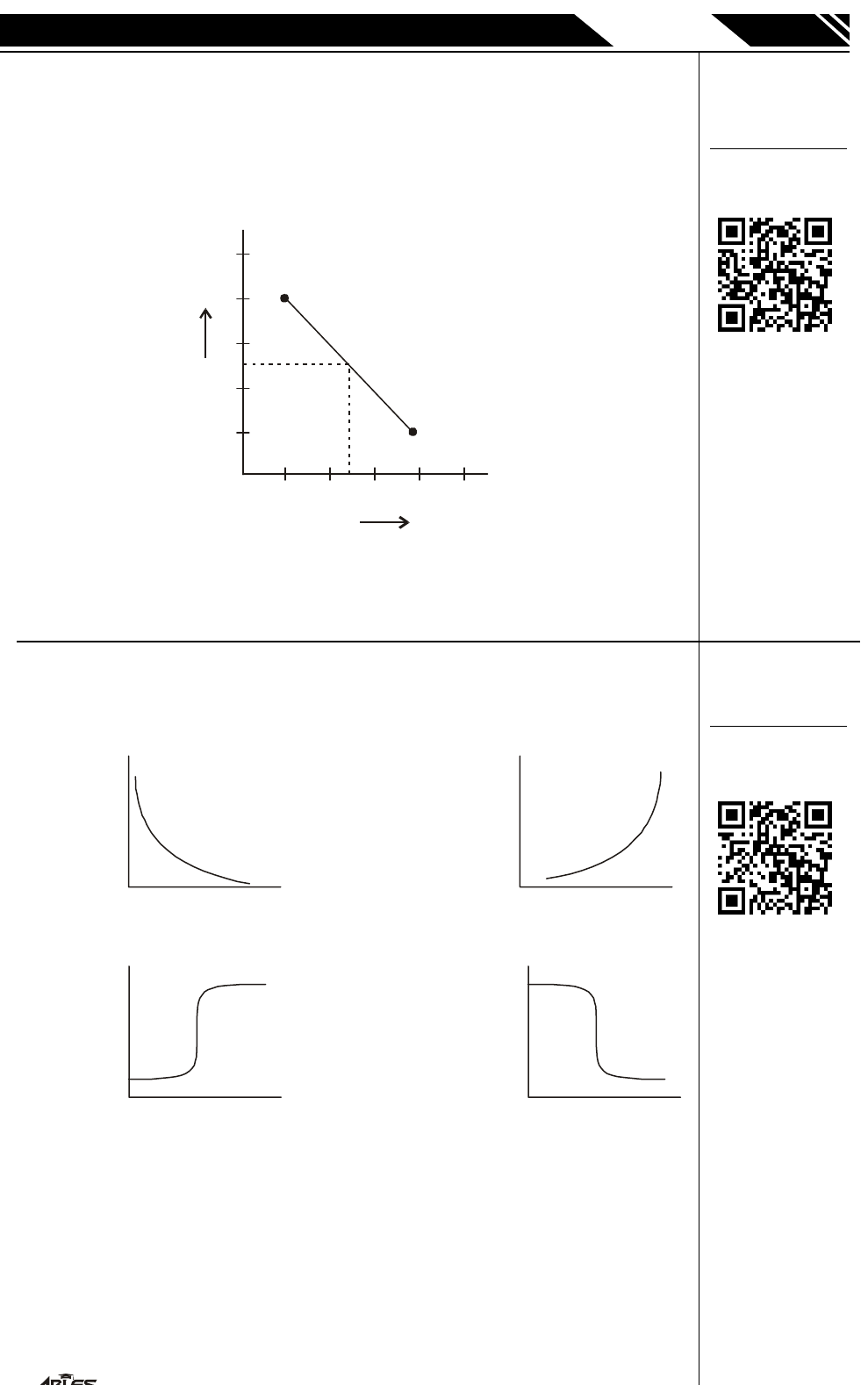IIT-JEE
CHEMISTRY
P. JOY
PROMOTIONAL MATTER
4
,
588-A, TAL
W
ANDI, KOT
A
(
Q.10 One more way to get from 'A' state 'A' to state 'B' is to
follow a direct path through state 'F'. This process is not
isobaric, isochoric, isothermal or adiabatic yet you can draw
some conclusions about its energetics using the first law of
thermodynamics. Which of the following are true for the
first half of the process-
1
1
2
3
A
P
V
B
F
4
5
2
3
4
5
(A) Both T and E increase
(B) Work energy flows in
(C) Q flows in
(D) Q is larger in magnitude than w
Q.11 Of the graphs below, the one that best illustrates the
relationship between [H
3
O
+
(aq)
] and [OH
(aq)
] in a solution
is-
(A)
[OH]
(aq)
[
H
O
]
3
(aq)
+
(B)
[OH]
(aq)
[
H
O
]
3
(aq)
+
(C)
[OH]
(aq)
[
H
O
]
3
(aq)
+
(D)
[OH]
(aq)
[
H
O
]
3
(aq)
+
To open video solution go to
https://ables.online/20video
or
Scan following QR Code
To open video solution go to
https://ables.online/20video
or
Scan following QR Code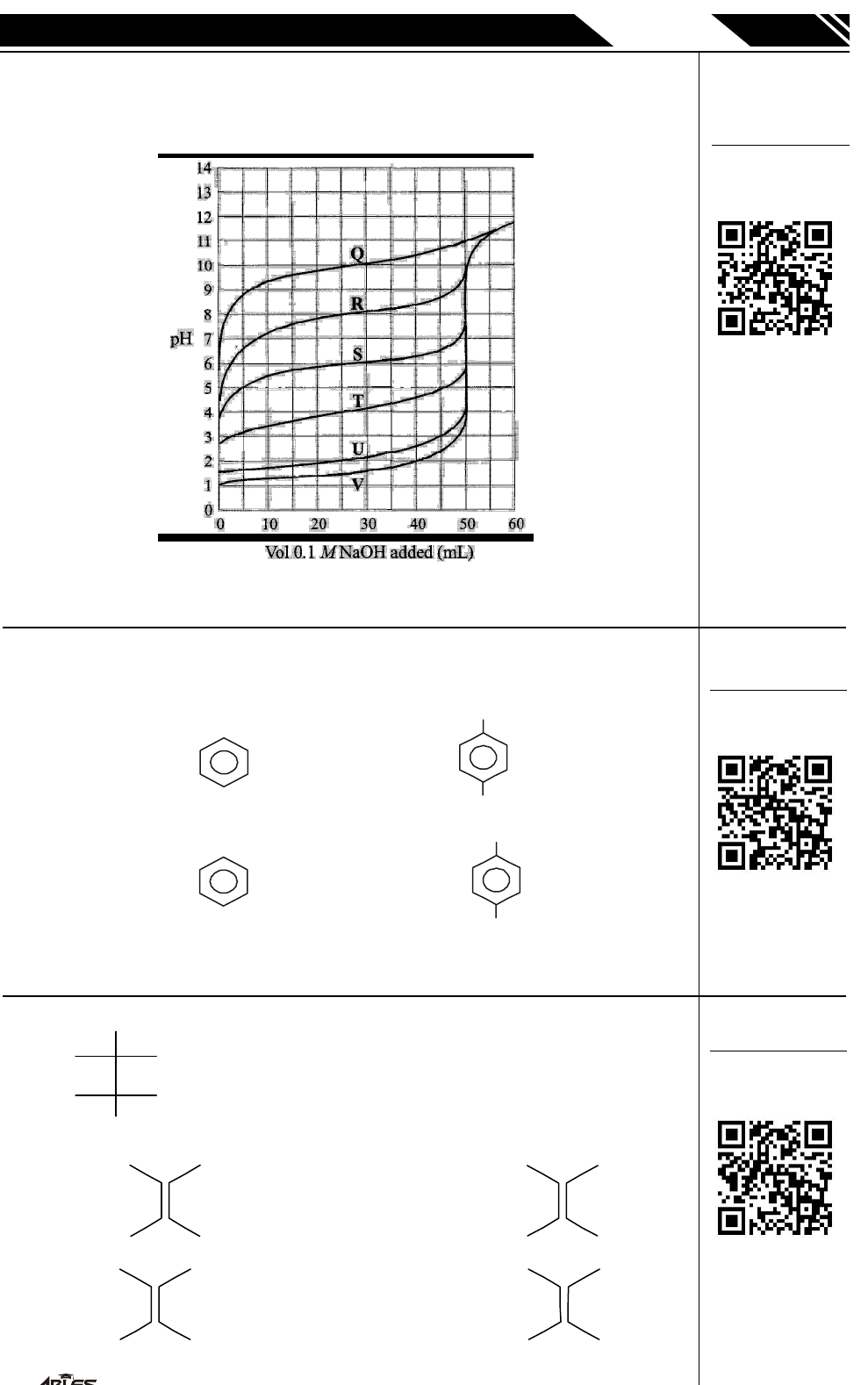IIT-JEE
CHEMISTRY
P. JOY
PROMOTIONAL MATTER
5
,
588-A, TAL
W
ANDI, KOT
A
(
Q.12 The graph below shows titration curves of 50 mL of the six
acids, Q, R, S, T, U, and V. In each case, the titrant used
wasO.lMNaOH.
What was the concentration of acid S before the titration?
(A) 0.05 M (B) 0.1 M (C) 0.2 M (D) 1M
Q.13 Which of the following statement is correct about reaction
1 & reaction 2.
Reaction 1 :
() /
() /h/.
() /FeBr
1
2
3
25 3
2
2 3
C H Cl AlCl
Br alc KOH
Br
¾ ®¾¾¾¾¾¾
n
Br
CH = CH
2
Reaction 2 :
() /
() /FeBr
() /h/.
1
2
3
25 3
2 3
2
C H Cl AlCl
Br
Br alc KOH
¾ ®¾¾¾¾¾¾
n
Br
CH = CH
2
(A) Only reaction 1 is correct (B) Only reaction 2 is correct
(C) Both reaction are correct (D) None of them are correct
Q.14
Br
Ph
Br
H
H
Ph
NaI
(acetone)
¾ ®¾ ¾¾
A, A is :
(A)
H
Ph
Ph
H
(B)
H
Ph
H
Ph
(C)
H
Ph
H
Br
(D)
H
Br
H
Br
To open video solution go to
https://ables.online/20video
or
Scan following QR Code
To open video solution go to
https://ables.online/20video
or
Scan following QR Code
To open video solution go to
https://ables.online/20video
or
Scan following QR Code